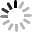## Most Practical Formulas of LED Display Summary!

December 28, 2022

The installation of Shenzhen Kingvisionled LED display screens usually needs to calculate some parameters such as spacing, height and width, numbers of modules, etc. Today we will present some common and also the most practicle calculation fomulas of LED display boards.

Table of Content

1. Viewing distance of LED display screen

2. Length and height

3. The number of LED display modules

4. LED lamp light spacing

5. Scanning mode

6. Brightness

7. Resolution## 1.Viewing distance of LED Display Screen

The viewing distance can be the appropriate distance that you can see the images on the LED screen clearly. Basically, we have the minimum viewing distance, optimum viewing distance and maximum viewing distance, and we list the calculation here. However, it is worth noting that the correct viewing distance not only relates to the pixel pitch, but also the total size of the LED screen and the specific condition.

(1) Minimum viewing distance (smooth image provided)

Pixel Pitch(mm) × 1000/1000 = Minimum Viewing Distance (m)

For example, a LED screen with 10mm pitch has a minimum viewing distance of 10 meters, and a 20mm pitch screen has a minimum viewing distance of 20 meters.

(2) Optimum viewing distance (HD image provided)

Pixel Pitch(mm) × 3000/1000 = Optimum Viewing Distance (m)

The optimum distance is three times longer than minimum viewing distance.

(3) Maximum viewing distance

Height × 30 = Maximum Viewing Distance (m)

For example, a LED screen with 2m height can be viewed from 60 meters away.

## 2.Length and Height

Spacing Distance × Dot Number = Length/Height

There we list two cases:

Case 1:

Length of P16 Modules = 16 (dot number) × 1.6cm = 25.6cm

Height of P16 Modules = 8 (dot number) × 1.6cm = 12.8cm

Case 2:

Length of P10 Modules = 32 (dot number) × 1.0cm = 32cm

Height of P10 Modules = 16 (dot number) × 1.0cm = 16cm

## 3.The Number of LED Display Modules

(1)Total Area ÷ Length of Modules ÷ Height of Modules = the Number of LED Modules

Case 1:

For 10 square meters of P16mm LED display, the number of LED display should be:

10㎡ ÷ 0.256m (length) ÷ 0.128m (width) = 305.17678pcs ≈ 305pcs

(2) For more accurate calculation:

Number of Modules for Length × Number of Modules for Height = Total Number of Modules

Case 2:

The number of LED modules for length euqals to 5m ÷ 0.256m (length of single module) = 19.53125pcs ≈ 20pcs

The number of LED modules for height euqals to 2m ÷ 0.128m (height of single module) = 15.625 ≈ 16pcs

So the total number of modules is 20pcs × 16pcs = 320pcs

## 4.LED Lamp Lights Spacing

The spacing measures the distance from one pixel point to that of the neighboring one, and it can be straightforward to understand. For example, the spacing distace of P16 LED display module is 16mm, for P20 module is 20mm and that of P12 LED screen module is 12mm.

## 5.Scanning mode

Before we calculate this parameter, let’s clear up the some definition of scan mode. In a certain display area, the ratio of the number of lines that are simultaneously lit to the number of lines in the entire area is called the scanning mode.

There are basically two scanning mode, they are static scanning and dynamic scanning. If the driving circuit lights up every pixel point of the LED display screen each time, this scan mode is called static sanning. And if the lighting up is discontinous, for example, there are 1, 2, 3, 4, 5, 6, 7, 8 rows of pixel points on the screen, and the driving circut only lights up 1 and 3 rows at the first time, and go out them and light up 3,5 rows in a very short time – this is what we call dynamic scanning, which has lower brightness compared with static scanning but can reduce the power consumption.

For example, if the pixel for the full color LED display module is 16 × 8 (2R1G1B), and use the the 16 bit MBI5026 to drive, then there will be 512/16 = 32.

(1) If use 32 MBI5026 chips, it is static scanning;

(2) If 16 MBI5026 chips, it is 1/2 dynamic scanning;

(3) If 8 MBI5026 chips, it is 1/4 dynamic scanning.## 6.Brightness

For a P16 LED display board with 2R1G1B LED lamp beads and 1/4 scan mode, there are 3906 dots on per square meter. If the brightness of red LED, green LED and blue LED are 800mcd, 2300mcd and 350mcd separately, the brightness for per square meter should be:

(800 × 2 + 2300 + 350) × 3906/1000/4=4150cd/㎡

The fomulas for calculating the brightness of pixel are (2R1G1B):

(1) Brightness for red LED: Brightness Per Square Meter/Dot Number Per Square Meter × 0.3/2

(2) Brightness for green LED: Brightness Per Square Meter/Dot Number Per Square Meter × 0.6

(3) Brightness for blue LED: Brightness Per Square Meter/Dot Number Per Square Meter × 0.1

For instance, the LED module has 2500 dot per square meter, 2R1G1B, and the brightness shall be 5000 cd/㎡:

Brightness of red LED: 5000 ÷ 2500 × 0.3 ÷ 2 = 0.3

Brightness of green LED: 5000 ÷ 2500 × 0.6 ÷ 2 = 1.2

Brightness of blue LED: 5000÷2500×0.1=0.2

The brightness of each pixel is: 0.3×2+1.2+0.2=2.0cd

## 7.Resolution

The Resolution Per Square Meter = 1/pixel pitch (m)/pixel pitch (m)

For example, resolution of P16 LED screen = 1/0.016/0.016 = 3906 point/㎡

You can easily understand there is a negative correlation between the pixel pitch and resolution, that is, the smaller the pixel pitch, the higher the resolution, and the better the image quality.